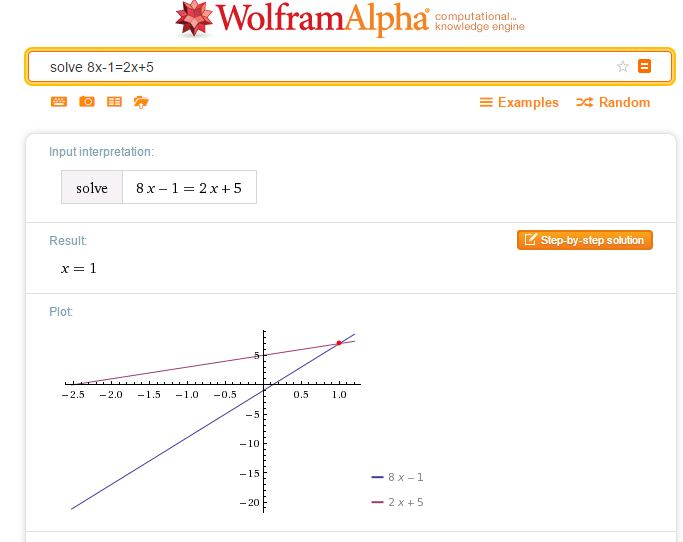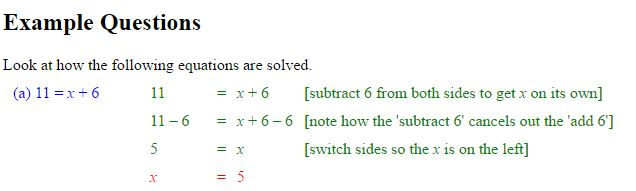# Low Stakes Testing in the Mathematics Classroom

Please note – a completely new version of this presentation with many more resources and additional ideas will be presented at the BCME Conference, April 2018. Following my sessions, I will update all relevant pages

Low Stakes Testing in the Mathematics Classroom (PowerPoint File, takes a few moments to download)

Or use this shorter version to see the slides on the student survey on low stakes tests.
Mini Tests Colleen Young for PowerPoint

Slides from the BERA Conference, Learning from the classroom – Practitioner research in mathematics education – July 2016

There are many hyperlinks provided in the presentation, for ease of access these are also provided below:

# Solving Linear Equations

A collection of resources to use to demonstrate and practise solving equations.

Student Exercises
I find Owen Elton’s worksheet, Balancing Equations on TES Resources very useful when introducing equations, the diagrams emphasise that we must do the same to both sides.
(See Diagrams in Mathematics, for more on the use of diagrams to help understanding).

As an alternative to the balance approach, consider this doing / undoing approach, described here by Don Steward, this is an approach I use for finding inverse functions. The exercises Don refers to are here.

One of the Standards Unit resources, A2 Creating and solving equations (in Mostly Algebra) uses this approach, students create an equation and  then undo it; this is a great exercise for demonstrating notation. Another resource with this approach comes from the Mathematics Assessment Project (the design and development was led by the MARS Shell Center team at the University of Nottingham) Building and Solving Linear Equations lesson

For a superb collection of ideas and student exercises for solving linear equations see all Don Steward’s posts tagged linear equations. Many of these outstanding resources use a very visual approach with very clear diagrams to help students’ understanding.

There are numerous questions on linear equations on Diagnostic Questions.A simple way to check a solution to an equation of any type is to simply enter your equation as a WolframAlpha query. Note that WolframAlpha includes a graphical illustration; it is so important for students to understand how equations may be solved graphically; I always illustrate graphical solutions when we are working with Algebra to help students make these links. Desmos of course, is ideal here.Centre for Innovation in Mathematics TeachingCIMT
have tutorials on equations: Linear Equations 1, Linear Equations 2, and Linear Equations with Brackets.

The following three resources work well for demonstrating the balance method of solving linear equations.

Duncan Keith’s Linear Equation Calculator is available on STEM Learning.Choose the type of equation you require then the sequence of operations required to solve the equation.
Select Do it after each operation, for example -32 Do it were the keys selected to start the above problem.

The slideshow below shows how to use the calculator to solve equations where the unknown is on both sides.

Mathisfun

Mathisfun has this very clear and easy to use interactive illustrating the solution of linear equations.

# Circles & Tangents

Use Desmos to explore tangents to circle through given point

Teaching Year 10 about the equation of a tangent to a circle at a given point I have created a Desmos page. I have also created a Diagnostic Questions Quiz using questions on circles (centre the origin) from Diagnostic Questions.
(pdf: quation of a Circle & Gradient of Tangent)

(Also added to GCSE New Content page)

An early introduction – plot some points and functions
Desmos – points & functions (for PowerPoint file)

Graphs-GCSE for PowerPoint file

# Desmos dot to dot

Continuing with my Desmos theme (see the excellent Activity Builder from last week), a happy discovery – you can join plotted points on Desmos. This is ideal for creating cumulative frequency graphs for example – see the example in the following slides.

For completeness, so all the information and examples on Desmos are in one place, I have added pages to the Desmos series.

# Desmos Activity Builder

A superb new addition – Desmos have created an activity builder for teachers, this enables the creation of interactive Desmos-based classroom activities. A series of pages can be created – any combination of graphs, questions or text. Having created and saved the activity a code is then associated with that  activity which your students enter at student.desmos.com.A simple experiment for my first activity – identify the lines x=k and y=k.
Activities are very easy to create, the interface is intuitive; images can very easily be added by dragging them to the page.

This slideshow requires JavaScript.

Once students have tried the activity and submitted answers to any questions a teacher can see all their submitted responses. This is certainly something I will be using with my students in the coming academic year. I have various slideshows for Desmos which I can now create activities for. (For more on Desmos, see these pages).